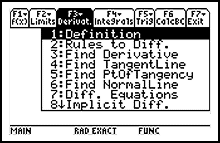# Software, OS updates and apps

## Calculus Made Easy for TI-89 TitaniumSolve differential equations, find derivatives, graph slope fields and check for concavity.

## Features Summary

Calculus Made Easy is the ultimate educational Calculus tool.

Users have boosted their calculus understanding and success by using this user-friendly product. A simple menu-based navigation system permits quick access to any desired topic.

This comprehensive application provides examples, tutorials, theorems, and graphical animations. Just enter the given function in the provided dialogue box and learn how the correct rule is applied - step by step - until the final answer is derived.

View samples online at www.ti89.com/slide.htm.

User feedback and sample screenshots can be found at www.ti89.com.

### Integration and Differentiation

Calculus Made Easy provides step-by-step solutions to integration and differentiation problems:

• U-Substitution
• Integration by Parts
• Partial Fractions
• Power Rule
• Expand/Rewrite
• Arctan(x)
• Arcsin(x)
• Integrals
• Chain, Power, Product and Quotient Rule

### Coursework Coverage

Calculus Made Easy covers four years of calculus:

• AP Calculus AB/Calc I: functions, limits, derivatives, integrals, diff-E-Q, trigonometry, motion, related rates, volume, area approximation, implicit differentiation
• AP Calculus BC/Calc II: parametric, polar, vector, advanced integrals, sequences and series
• Multivariable Calculus/Calc III
• Vector Calculus
• Differential Geometry

### Functions Module

In the Functions module, the Function Explorer allows users to find the properties of a function. Students can:

• Find the domain or range of a function
• Find the composition of two functions
• Find the inverse function
• Vertical, horizontal or slant asymptotes
• Assess symmetry
• Locate zeros and critical points
• Find the absolute and relative extrema and
• Compute points of inflection

### AP Calculus AB Module

• Find limits, derivatives, anti-derivatives, tangent and normal lines
• Find the derivative of an inverse function
• Graph an equation
• Find the symbolic first and second derivative
• Check slope/concavity at a point

### Trigonometry

• Trig-identities
• Derivatives
• Integrals
• Natural log